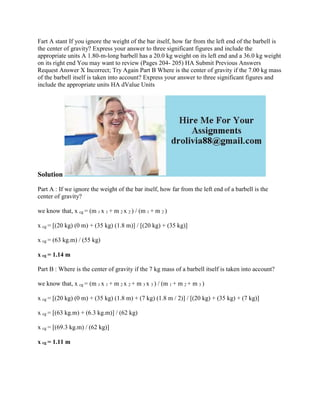Se está descargando tu SlideShare. ×

# Fart A stant If you ignore the weight of the bar itself- how far from.docx

Anuncio
Anuncio
Anuncio
Anuncio
Anuncio
Anuncio
Anuncio
Anuncio
Anuncio
Anuncio
AnuncioCargando en…3
×

1 de 2 Anuncio

# Fart A stant If you ignore the weight of the bar itself- how far from.docx

Fart A stant If you ignore the weight of the bar itself, how far from the left end of the barbell is the center of gravity? Express your answer to three significant figures and include the appropriate units A 1.80-m-long barbell has a 20.0 kg weight on its left end and a 36.0 kg weight on its right end You may want to review (Pages 204- 205) HA Submit Previous Answers Request Answer X Incorrect; Try Again Part B Where is the center of gravity if the 7.00 kg mass of the barbell itself is taken into account? Express your answer to three significant figures and include the appropriate units HA dValue Units
Solution
Part A : If we ignore the weight of the bar itself, how far from the left end of a barbell is the center of gravity?
we know that, x cg = (m 1 x 1 + m 2 x 2 ) / (m 1 + m 2 )
x cg = [(20 kg) (0 m) + (35 kg) (1.8 m)] / [(20 kg) + (35 kg)]
x cg = (63 kg.m) / (55 kg)
x cg = 1.14 m
Part B : Where is the center of gravity if the 7 kg mass of a barbell itself is taken into account?
we know that, x cg = (m 1 x 1 + m 2 x 2 + m 3 x 3 ) / (m 1 + m 2 + m 3 )
x cg = [(20 kg) (0 m) + (35 kg) (1.8 m) + (7 kg) (1.8 m / 2)] / [(20 kg) + (35 kg) + (7 kg)]
x cg = [(63 kg.m) + (6.3 kg.m)] / (62 kg)
x cg = [(69.3 kg.m) / (62 kg)]
x cg = 1.11 m
.

Fart A stant If you ignore the weight of the bar itself, how far from the left end of the barbell is the center of gravity? Express your answer to three significant figures and include the appropriate units A 1.80-m-long barbell has a 20.0 kg weight on its left end and a 36.0 kg weight on its right end You may want to review (Pages 204- 205) HA Submit Previous Answers Request Answer X Incorrect; Try Again Part B Where is the center of gravity if the 7.00 kg mass of the barbell itself is taken into account? Express your answer to three significant figures and include the appropriate units HA dValue Units
Solution
Part A : If we ignore the weight of the bar itself, how far from the left end of a barbell is the center of gravity?
we know that, x cg = (m 1 x 1 + m 2 x 2 ) / (m 1 + m 2 )
x cg = [(20 kg) (0 m) + (35 kg) (1.8 m)] / [(20 kg) + (35 kg)]
x cg = (63 kg.m) / (55 kg)
x cg = 1.14 m
Part B : Where is the center of gravity if the 7 kg mass of a barbell itself is taken into account?
we know that, x cg = (m 1 x 1 + m 2 x 2 + m 3 x 3 ) / (m 1 + m 2 + m 3 )
x cg = [(20 kg) (0 m) + (35 kg) (1.8 m) + (7 kg) (1.8 m / 2)] / [(20 kg) + (35 kg) + (7 kg)]
x cg = [(63 kg.m) + (6.3 kg.m)] / (62 kg)
x cg = [(69.3 kg.m) / (62 kg)]
x cg = 1.11 m
.

Anuncio
Anuncio

### Fart A stant If you ignore the weight of the bar itself- how far from.docx

1. 1. Fart A stant If you ignore the weight of the bar itself, how far from the left end of the barbell is the center of gravity? Express your answer to three significant figures and include the appropriate units A 1.80-m-long barbell has a 20.0 kg weight on its left end and a 36.0 kg weight on its right end You may want to review (Pages 204- 205) HA Submit Previous Answers Request Answer X Incorrect; Try Again Part B Where is the center of gravity if the 7.00 kg mass of the barbell itself is taken into account? Express your answer to three significant figures and include the appropriate units HA dValue Units Solution Part A : If we ignore the weight of the bar itself, how far from the left end of a barbell is the center of gravity? we know that, x cg = (m 1 x 1 + m 2 x 2 ) / (m 1 + m 2 ) x cg = [(20 kg) (0 m) + (35 kg) (1.8 m)] / [(20 kg) + (35 kg)] x cg = (63 kg.m) / (55 kg) x cg = 1.14 m Part B : Where is the center of gravity if the 7 kg mass of a barbell itself is taken into account? we know that, x cg = (m 1 x 1 + m 2 x 2 + m 3 x 3 ) / (m 1 + m 2 + m 3 ) x cg = [(20 kg) (0 m) + (35 kg) (1.8 m) + (7 kg) (1.8 m / 2)] / [(20 kg) + (35 kg) + (7 kg)] x cg = [(63 kg.m) + (6.3 kg.m)] / (62 kg) x cg = [(69.3 kg.m) / (62 kg)] x cg = 1.11 m